# Rewrite the equation below in slope intercept form y = mx + b

Rewrite the equation below in slope intercept form y = mx + b. Use fractions for the slope and the y-intercept, as needed.
4x+5y=7

I will be happy to critique your work. Do exactly what it says in the first sentence.

x - y + 1=0

source: math

#### Earn Coin

Coins can be redeemed for fabulous gifts.

Similar Homework Help Questions
• ### Rewrite the given equation in slope intercept form y = mx + b

Rewrite the given equation in slope intercept form y = mx + b. Use fractions for the slope and the y-intercept, as needed. 4/5x + 6/5y=4

• ### Rewrite the equation below in slope intercept form y = mx + b

Rewrite the equation below in slope intercept form y = mx + b. Use fractions for the slope and the y-intercept, as needed. 4x+7y=9 Rewrite the equation below in general form Ax + By = C where A, B and C are the smallest integers possible and A > 0. Enter the variable expression Ax + By separately from the constant term C. y=4/7x+2/17 Write an equation of the line that passes through the point (-6, 7) and is parallel...

• ### a. Rewrite the given equation in slope-intercept form by solving for y b. Give the slope...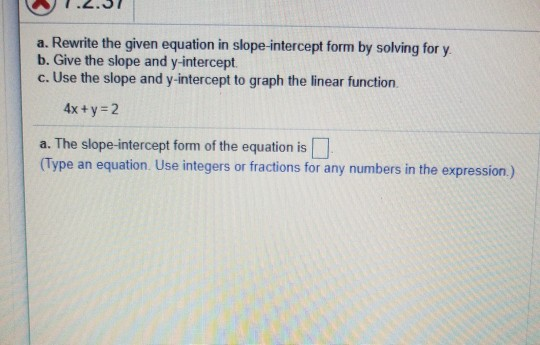a. Rewrite the given equation in slope-intercept form by solving for y b. Give the slope and y-intercept c. Use the slope and y-intercept to graph the linear function. 4x +y 2 a. The slope-intercept form of the equation is (Type an equation. Use integers or fractions for any numbers in the expression.)

• ### Write the following equation in slope-intercept form and identify the slope and y-intercept. 5x-2y 3 The...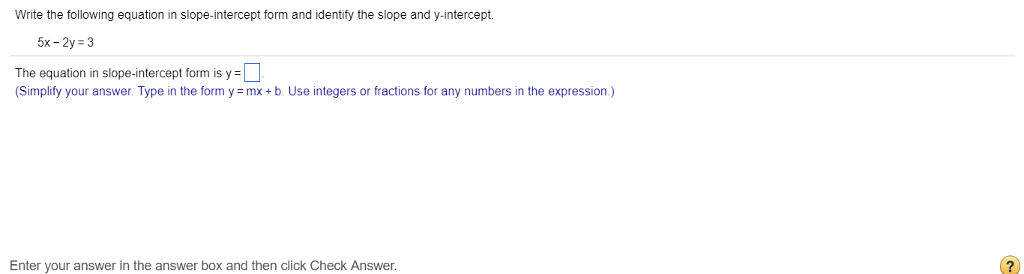Write the following equation in slope-intercept form and identify the slope and y-intercept. 5x-2y 3 The equation in slope-intercept form is y- (Simplify your answer. Type in the form y mx+b. Use integers or fractions for any numbers in the expression.) Enter your answer in the answer box and then click Check Answer.

• ### Write an equation for line L in point-slope form and slope-intercept form. L is parallel to...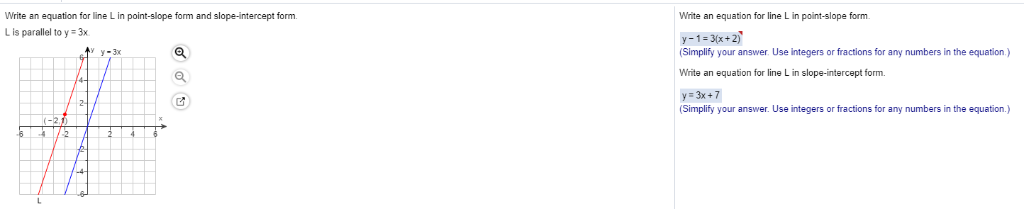Write an equation for line L in point-slope form and slope-intercept form. L is parallel to y 3x Write an equation for line L in point-slope form y-1-3(x+2) (Simplify your answer. Use integers or fractions for any numbers in the equation.) Write an equation for line L in slope-intercept form. y-3x Simplify your answer. Use integers or fractions for any numbers in the equation.])

• ### There is a question > Write the slope intercept form of the equation for the line passing through the points (-6,3) and (0,7) Now my answer started with > Slope = 7-3/0-(-6) = Slope = 2/3 Then to find the intercept > Y=mx+c 3= 2/3(-6) +

There is a question >Write the slope intercept form of the equation for the line passing through the points (-6,3) and (0,7) Now my answer started with >Slope = 7-3/0-(-6) = Slope = 2/3Then to find the intercept > Y=mx+c 3= 2/3(-6) + cc= 7In my opinion this is the only way I could think of besides the formula y0-y1=m(x0-x1) and I tried that and I am getting nothing..So where am I going wrong?The right answer according to mathlab is...

• ### Solve for the unknown value in the following equation, y=mx+b, given that the slope and intercept...

Solve for the unknown value in the following equation, y=mx+b, given that the slope and intercept of the line are 16.567 and 0.224, respectively, and that the y value of your ordered pair is 5.2

• ### equation 4x = 5y + 7

equation 4x = 5y + 7. You want to find a line that has that point and is parallel to that line. You must first convert that equation into the y = mx + b form. 5y = 4x - 7; y = 4x/5 - 7/5; we know that a parallel line has a slope that is the same which would be 4/5. Do you know how to find the y intercept

• ### Use the given conditions to write an equation for the line in point-slope form and slope-intercept...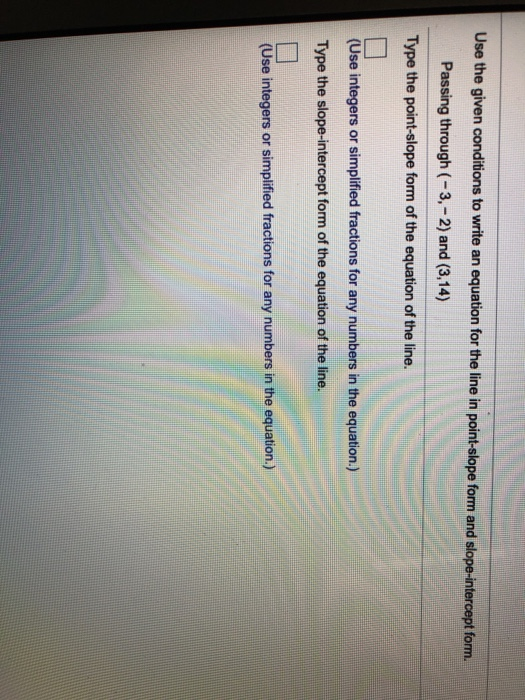Use the given conditions to write an equation for the line in point-slope form and slope-intercept form. Passing through (-3,-2) and (3,14) Type the point-slope form of the equation of the line. (Use integers or simplified fractions for any numbers in the equation.) Type the slope-intercept form of the equation of the line. (Use integers or simplified fractions for any numbers in the equation.)

• ### Use the given conditions to write an equation for the line in point-slope form and slope-intercept...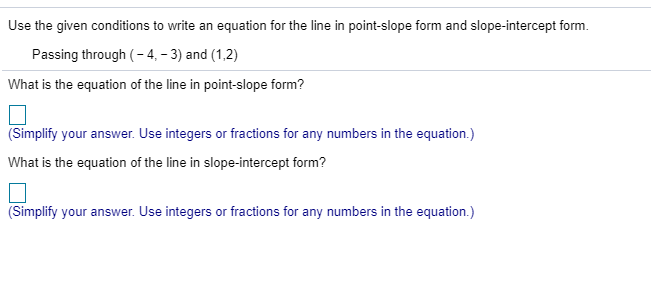Use the given conditions to write an equation for the line in point-slope form and slope-intercept form. Passing through (-4,-3) and (1,2) What is the equation of the line in point-slope form? (Simplify your answer. Use integers or fractions for any numbers in the equation.) What is the equation of the line in slope-intercept form? (Simplify your answer. Use integers or fractions for any numbers in the equation.)